# Chaos game

Martin McBride, 2020-09-06
Tags chaos game sierpinski triangle
Categories generativepy generative art

generativepy has moved to the pythoninformer.com website, and this page might not be up to date. Please visit the new location.

A chaos game is a simple system for generating an approximation to a particular fractal. It relies on a collection of rules that transform one point (x, y) into a different point (x', y').

The game proceeds like this:

1. Pick an initial point (x, y)
2. Choose one of the rules at random to generate (x', y')
3. Replace the current (x, y) with the transformed (x', y')
4. Mark the pixel at (x, y)
5. Repeat from step 2, for a fixed number of iterations. picking P at random each time

## Creating a Sierpinski triangle

Creating a Sierpinski triangle is quite simple using this method.

We first define 3 points, A, B and C that make up the corners of the triangle. For an equilateral triangle with sides of length 2, centred on the origin, the coordinates of the vertices are shown below (0.866 is the sine of 60 degrees):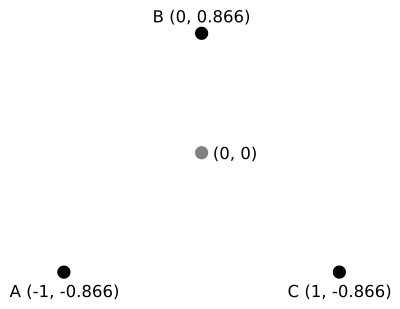The process then works as follows:

1. Pick an initial point (x, y) that is within the triangle - (0, 0) works well
2. Choose one of the points A, B or C at random. (x', y') is the point halfway between the existing (x, y) and the chosen vertex
3. Replace the current (x, y) with the transformed (x', y')
4. Mark the pixel at (x, y)
5. Repeat from step 2, for a fixed number of iterations

Here are the first three iterations: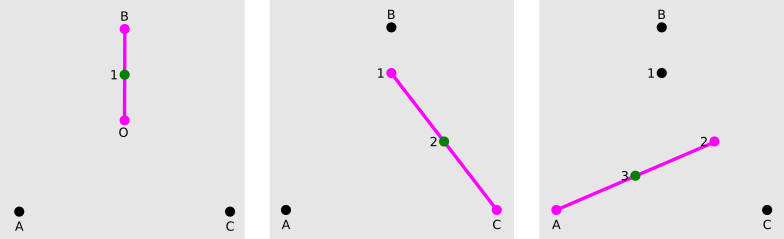Iteration 1 - let's suppose the first randomly selected vertex is B. We can then draw point 1 halfway between B and the initial point (0, 0).

Iteration 2 - suppose the next randomly selected vertex is C. We can then draw point 2 halfway between C and the previous point 1.

Iteration 3 - suppose the next randomly selected vertex is A. We can then draw point 3 halfway between A and the previous point 2.And so on...

At this point, it is easier to do things in code. After 1000 iterations things are just beginning to take shape:If you continue for 1,000,000 iterations you get something resembling a Sierpinski triangle: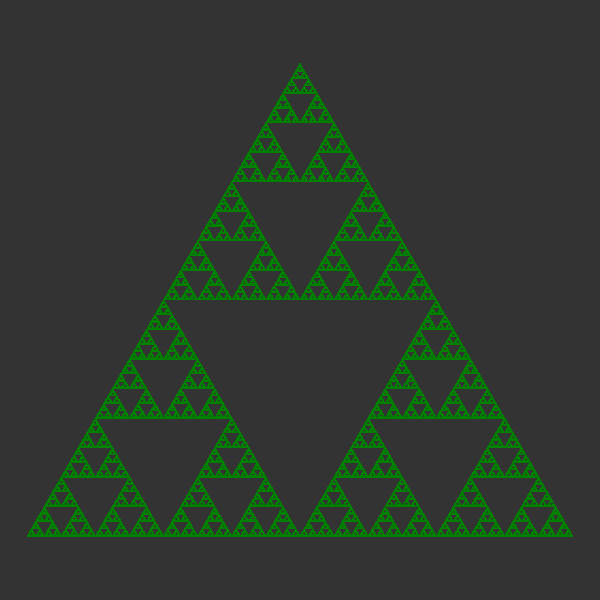Note that this is only an approximation to the triangle. But this technique can be used to create some very interesting patterns.

## The code

Since this code works by setting individual pixels, rather than drawing shapes, we use the make_bitmap function of generativepy. This function doesn't use Pycairo, instead it uses PIL, a Python bitmap image library.

Here is the code:

from generativepy.bitmap import make_bitmap, Scaler
from generativepy.color import Color
from PIL import ImageDraw
import math
import random

SIN60 = math.sin(math.pi/3)

SIZE = 600
RANGE = 1.1
ITERATIONS = 1000000
VERTICES = [(-1, SIN60),
(0, -SIN60),
(1, SIN60)]

def paint(image, pixel_width, pixel_height, frame_no, frame_count):
scaler = Scaler(pixel_width, pixel_height,
width=RANGE*2, height=RANGE*2,
startx=-RANGE, starty=-RANGE)
draw = ImageDraw.Draw(image)
draw.rectangle((0, 0, pixel_width, pixel_height),
fill=Color(0.2).as_rgbstr())
x, y = 0, 0
color = Color('green').as_rgbstr()
for i in range(ITERATIONS):
vertex = random.choice(VERTICES)
x = (x + vertex)/2
y = (y + vertex)/2
u, v = scaler.user_to_device(x, y)
draw.point((u, v), color)

make_bitmap("chaos-game-sierpinski.png", paint, SIZE, SIZE)


Taking the make_bitmap call first, we create a square image 600 pixels square. make_bitmap calls our paint function. It passes an empty PIL image of the correct size.

In the paint function we create a Scaler objects. The scaler object lets us convert between pixel positions and user coordinates. We set the usr coordinates to extend from -1.1 to +1.1 is both directions, so our triangle will fit nicely. User coordinates allow us to work in our chosen dimensions regardless of the actual pixel size of the image.

In order to be able to draw on the image we create a PIL Draw object. We also draw an initial rectangle that covers the entire image, and fill it with a dark grey (grey value 0.2) that serves as the image background.

Now the housekeeping is out of the way, the first part of the paint function sets things up:

    x, y = 0, 0
color = Color('green').as_rgbstr()


Our initial point (x, y) is (0, 0).

We create a green colour to draw the image pixels. PIL uses strings to specify colours. The as_rgbstr function converts a colour to a string of the form rgb(0, 128, 0) which is exactly what PIL needs.

Next we have the main loop:

    for i in range(ITERATIONS):
vertex = random.choice(VERTICES)
x = (x + vertex)/2
y = (y + vertex)/2
u, v = scaler.user_to_device(x, y)
draw.point((u, v), color)


We iterate 1,000,000 times. Each time through we:

• pick a random vertex
• calculate the point halfway between the chosen vertex and the previous point (x, y)
• convert the point (x, y), which is in user coordinates into a pixel position (u, v). The function scaler.user_to_device does this. The result is a pair of integer values that select a particular pixel
• We set the pixel at (u, v) to green using the point function of the draw object we created earlier. The point function sets a single pixel.

## Chaos game with a square

You might wonder what sort of fractal might we get if we used a square instead of a triangle?

It is easy enough to try, we could simply replace VERTICES with the four corners of a square. Unfortunately the results are disappointing. Instead of a fractal, you get a square full of random noise.

This improve if you add exclusions. For example, we could make an additional rule that when you pick a random vertex, it isn't allowed to be the same one you used last time. So if you used vertex B previously, you must choose between A, C or D next time. Here is the result: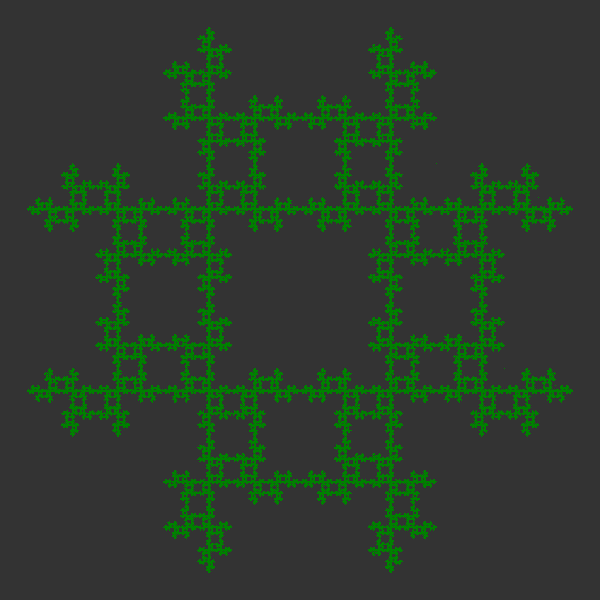And here is the Python code:

from generativepy.bitmap import make_bitmap, Scaler
from generativepy.color import Color
from PIL import ImageDraw
import math
import random

SIN60 = math.sin(math.pi/3)
COS60 = math.cos(math.pi/3)

SIZE = 600
RANGE = 1.1
ITERATIONS = 1000000
VERTICES = [(-1, -1),
(-1, 1),
(1, 1),
(1, -1)]
ALLOWED = [0, 1, 1, 1]
COUNT = len(VERTICES)

def paint(image, pixel_width, pixel_height, frame_no, frame_count):
scaler = Scaler(pixel_width, pixel_height,
width=RANGE*2, height=RANGE*2,
startx=-RANGE, starty=-RANGE)
draw = ImageDraw.Draw(image)
draw.rectangle((0, 0, pixel_width, pixel_height),
fill=Color(0.2).as_rgbstr())
x, y = 0, 0
oldn = 0
color = Color('green').as_rgbstr()
for i in range(ITERATIONS):
n = random.randrange(COUNT)
diff = (n - oldn) % COUNT
if ALLOWED[diff]:
vertex = VERTICES[n]
x = (x + vertex)/2
y = (y + vertex)/2
u, v = scaler.user_to_device(x, y)
draw.point((u, v), color)
oldn = n

make_bitmap("chaos-game-square.png", paint, SIZE, SIZE)


Here we have changed the VERTICES to be the four corners of a square. The ALLOWED list indicates which vertices are permitted. The list starts at the previous vertex and cycles round. So for example if the previous vertex was B, the allowed list [0, 1, 1, 1] would refer to vertices B, C, D, A. So the previous vertex B would be excluded, all others allowed.

Here is the main loop:

    for i in range(ITERATIONS):
n = random.randrange(COUNT)
diff = (n - oldn) % COUNT
if ALLOWED[diff]:
vertex = VERTICES[n]
x = (x + vertex)/2
y = (y + vertex)/2
u, v = scaler.user_to_device(x, y)
draw.point((u, v), color)
oldn = n


In this case we pick a number n in the range 0 to COUNT-1 (COUNT is the number of vertices). This represents the potential next vertex.

We calculate diff as the difference between n and the previous value, oldn, modulo COUNT. This serves as the index into the ALLOWED list.

We check if the current vertex is allowed. If not, we skip this iteration. If the vertex is allowed, we calculate the new vertex as before.

## Chaos game with a hexagon

We can change the square to a hexagon with the following code modifications:

SIN60 = math.sin(math.pi/3)
COS60 = math.cos(math.pi/3)

VERTICES = [(-1, 0),
(-COS60, SIN60),
(COS60, SIN60),
(1, 0),
(COS60, -SIN60),
(-COS60, -SIN60)]
ALLOWED = [0, 1, 0, 1, 0, 1]


The other code from the square example is unchanged. This uses a hexagon shape and excludes the same vertex from being used twice. Here is the result: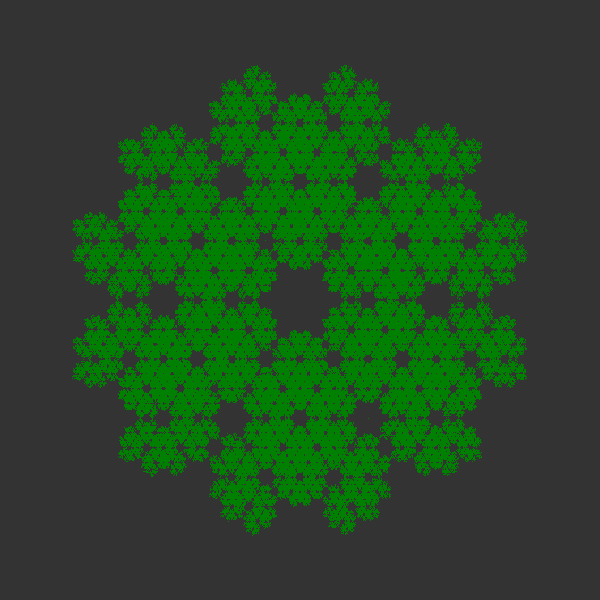If we use a different ALLOWED list [0, 1, 0, 1, 0, 1], then after using a particular vertex, the next vertex will have to be either the opposite or one of the two adjacent vertices. This is the result: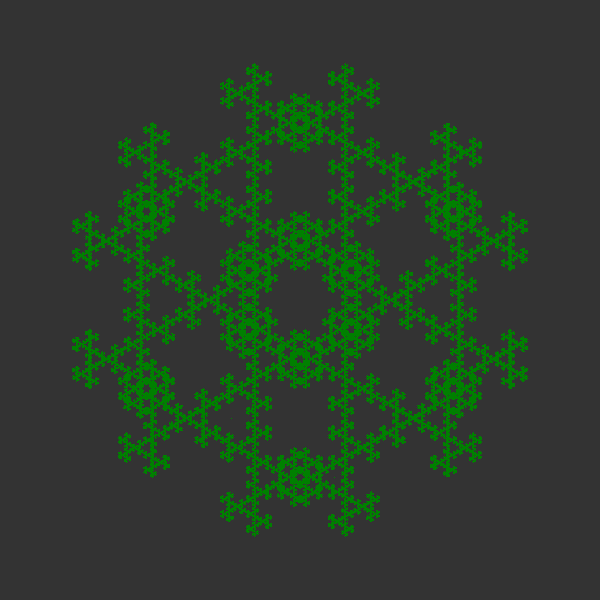You can try other shapes and allowed lists - you can also try irregular polygons.

Copyright (c) Axlesoft Ltd 2021